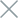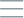• Home
• Tags
• Series
•# Heuristic Search Algorithm in Java

The Heuristic Search algorithm is an intelligent search method in Java programming that relies on using estimated information (knowledge) to guide the search process. Heuristics is an approximate method of problem-solving based on imperfect knowledge and estimated information about the current state of the problem.

## How Heuristic Search Algorithm Works

The Heuristic Search algorithm employs heuristic functions to evaluate the "closeness" of a state to the goal. During each search iteration, the algorithm selects a search direction based on the heuristic values of potential states. The goal is to optimize the heuristic value, leading to an approximate solution for the problem.

• Intelligent search: The algorithm uses estimated knowledge to guide the search, optimizing time and resources.
• Wide applicability: Heuristics can be applied to various optimization and search problems in real-world scenarios.

• Potential inaccuracy: Heuristics rely on estimation and potentially inaccurate information, resulting in imperfect solutions.

## Example and Explanation

A common example of the Heuristic Search algorithm is the A* algorithm, used for finding the shortest path on a map. Let's see how this algorithm works:

``````import java.util.*;

class Node {
int x, y;
int cost, heuristic;

Node(int x, int y, int cost, int heuristic) {
this.x = x;
this.y = y;
this.cost = cost;
this.heuristic = heuristic;
}
}

public class HeuristicSearchExample {
static int heuristic(int x, int y, int targetX, int targetY) {
return Math.abs(targetX - x) + Math.abs(targetY - y);
}

static void heuristicSearch(int[][] grid, int startX, int startY, int targetX, int targetY) {
PriorityQueue<Node> pq = new PriorityQueue<>((a, b) -> (a.cost + a.heuristic) - (b.cost + b.heuristic));
pq.offer(new Node(startX, startY, 0, heuristic(startX, startY, targetX, targetY)));

while (!pq.isEmpty()) {
Node current = pq.poll();
int x = current.x;
int y = current.y;

if (x == targetX && y == targetY) {
System.out.println("Found target at (" + x + ", " + y + ")");
return;
}

// Explore neighboring nodes and add to the priority queue
// based on total cost and heuristic
// ...
}
}
}
``````

In the example above, we use the A* algorithm to find the shortest path on a map. Neighboring nodes are explored based on the total cost to the current node and the heuristic estimation. The result is finding the shortest path from the starting point to the target point.